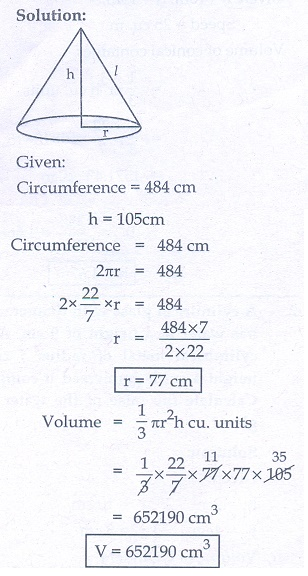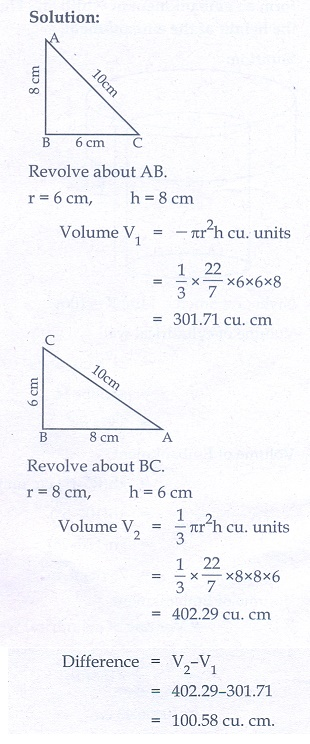Home | | Maths 10th Std | Exercise 7.2: Volume

# Exercise 7.2: Volume

Volume of a solid right circular cylinder, Volume of a hollow cylinder (volume of the material used), Volume of a right circular cone, Volume of sphere/hemisphere, Volume of frustum of a cone. (Maths Book back answers and solution for Exercise questions)

### Exercise 7.2

1. A 14 m deep well with inner diameter 10 m is dug and the earth taken out is evenly spread all around the well to form an embankment of width 5 m. Find the height of the embankment.2. A cylindrical glass with diameter 20 cm has water to a height of 9 cm. A small cylindrical metal of radius 5 cm and height 4 cm is immersed it completely. Calculate the raise of the water in the glass?3. If the circumference of a conical wooden piece is 484 cm then find its volume when its height is 105 cm.4. A conical container is fully filled with petrol. The radius is 10m and the height is 15 m. If the container can release the petrol through its bottom at the rate of 25 cu. meter per minute, in how many minutes the container will be emptied. Round off your answer to the nearest minute.5. A right angled triangle whose sides are 6 cm, 8 cm and 10 cm is revolved about the sides containing the right angle in two ways. Find the difference in volumes of the two solids so formed.6. The volumes of two cones of same base radius are 3600 cm3 and 5040 cm3. Find the ratio of heights.7. If the ratio of radii of two spheres is 4:7, find the ratio of their volumes.8. A solid sphere and a solid hemisphere have equal total surface area. Prove that the ratio of their volume is 3√3:4.9. The outer and the inner surface areas of a spherical copper shell are 576π cm2 and 324 π cm2 respectively. Find the volume of the material required to make the shell.10. A container open at the top is in the form of a frustum of a cone of height 16 cm with radii of its lower and upper ends are 8 cm and 20 cm respectively. Find the cost of milk which can completely fill a container at the rate of ₹40 per litre.1. 4.67 m

2. 1 cm

3. 652190 cm3

4. 63 minutes (approx)

5. 100.58

6. 5:7

7. 64:343

9. 4186.29 cm3

10. ₹ 418.36

Tags : Problem Questions with Answer, Solution | Mensuration | Mathematics , 10th Mathematics : UNIT 7 : Mensuration
Study Material, Lecturing Notes, Assignment, Reference, Wiki description explanation, brief detail
10th Mathematics : UNIT 7 : Mensuration : Exercise 7.2: Volume | Problem Questions with Answer, Solution | Mensuration | Mathematics